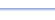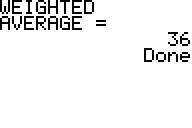Program: WTDAVG

WTDAVG

• Computes the weighted average, given the number of items and average in each group.

Useful for:

• SAT
• ACT
• Algebra 1
• Algebra 2
• Statistics

See full list of programs

Example

Q:   The average of four numbers is 44.  The average of five other numbers is 35.  The average of three other numbers is 27.  What is the average of all twelve numbers?

Solution:
Run WTDAVG.  How?

Enter 3 for the number of groups (A, B, C).
Average for group 1  = 44. Number of items in group 1 = 4.
Average for group 2  = 35. Number of items in group 2 = 5.
Average for group 3  = 27. Number of items in group 3 = 3.

The program reports that the weighted average is 36.

Interpretation: The average is 36.